MEDIUM
DATA STRUCTURES AND ALGORITHMS

# How to Build a Max Heap From an Array

HEAPSTREES
Written ByTom WagnerChristopher Ang

## What is a Max Heap?

A max heap is a type of binary tree stored in an array that is organized such that the maximum value is always at the root of the tree.

## Max Heap Examples

Given an array of integers, transform the array in-place to a max heap.

### Example 1

Input: [1,2,3,4,5,6,7]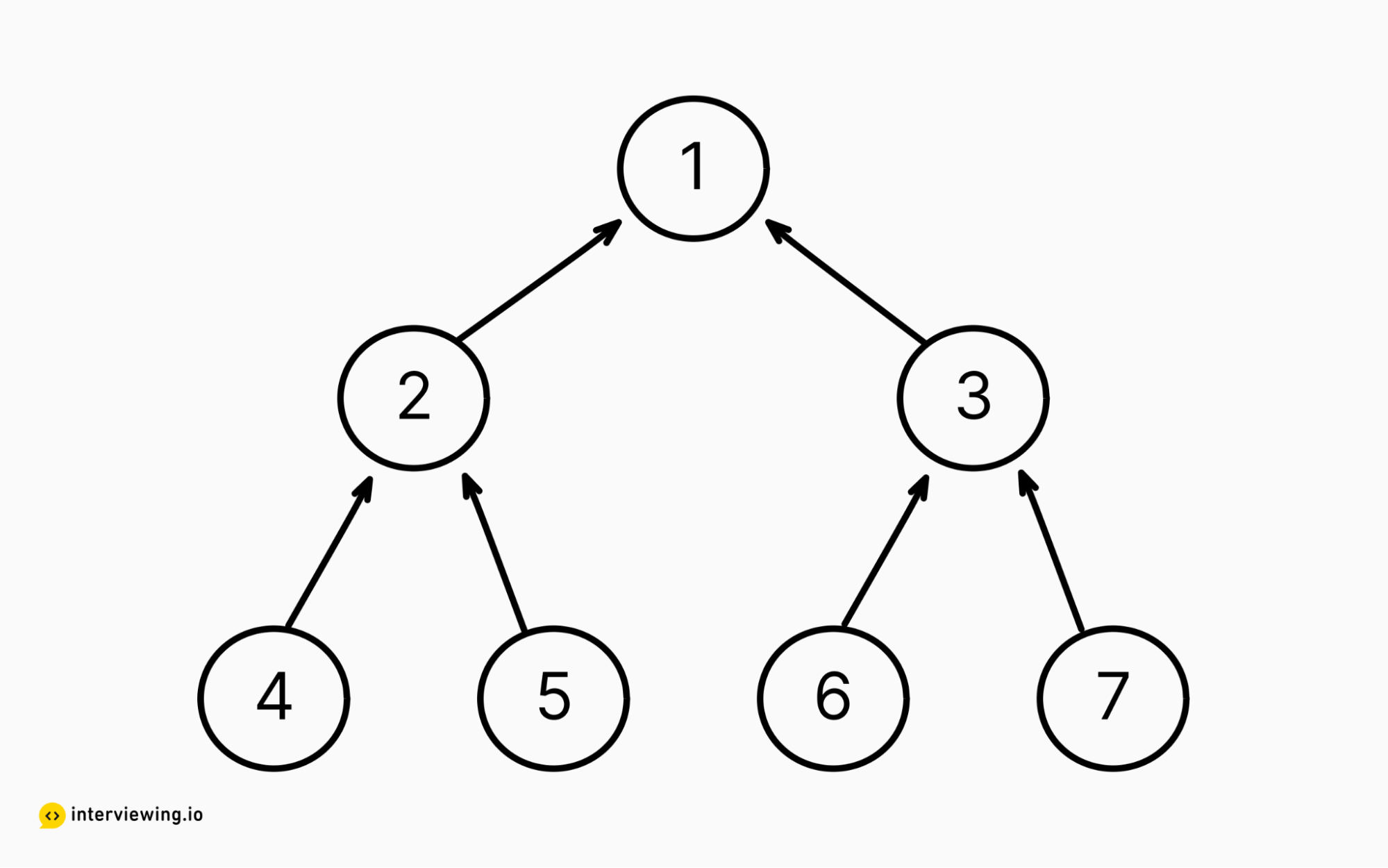Output:

• [7,4,6,1,3,2,5] (sift up)

OR

• [7,5,6,4,2,1,3] (sift down)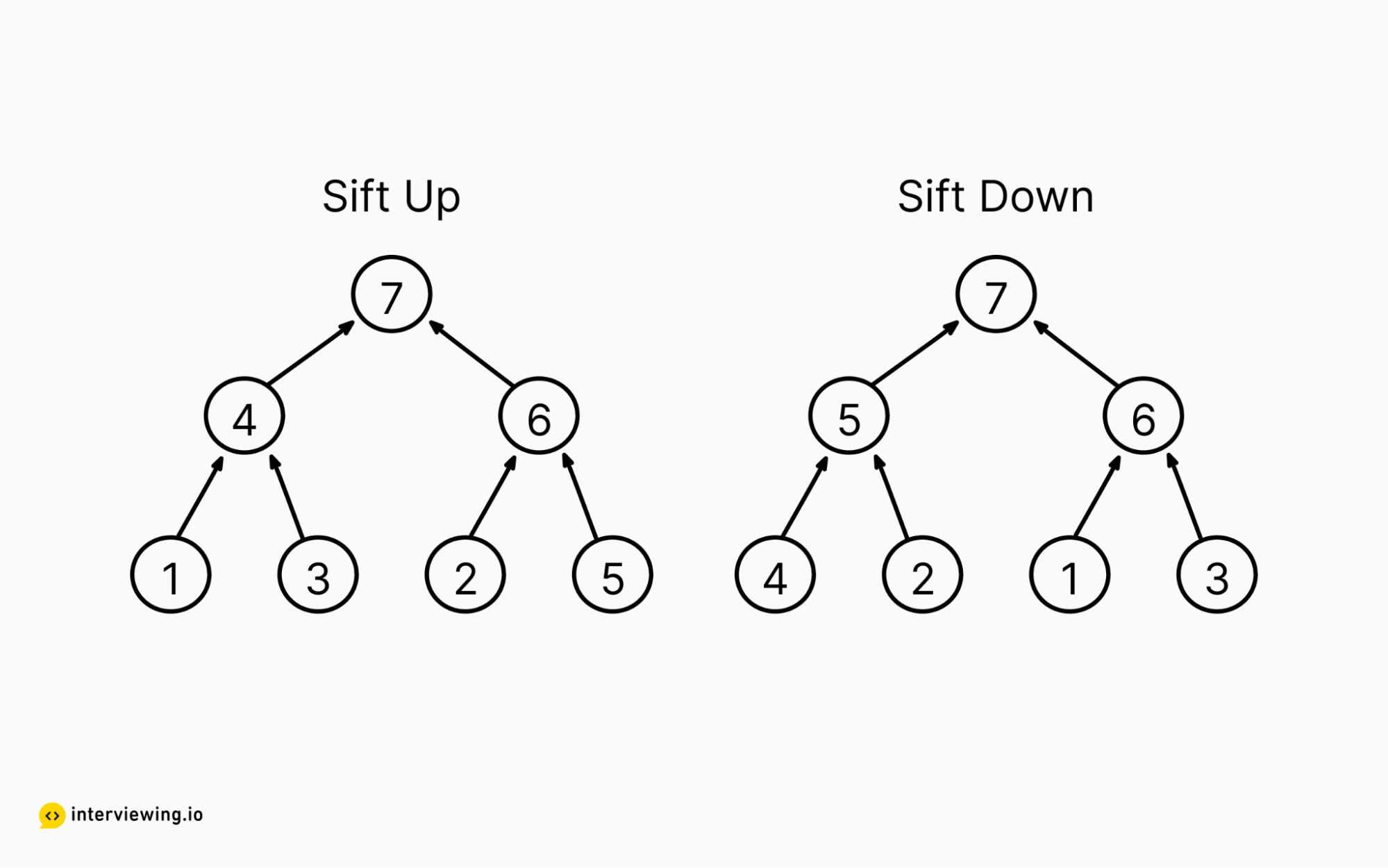### Example 2

Input: [4,6,52,10,17,28,39,43,11]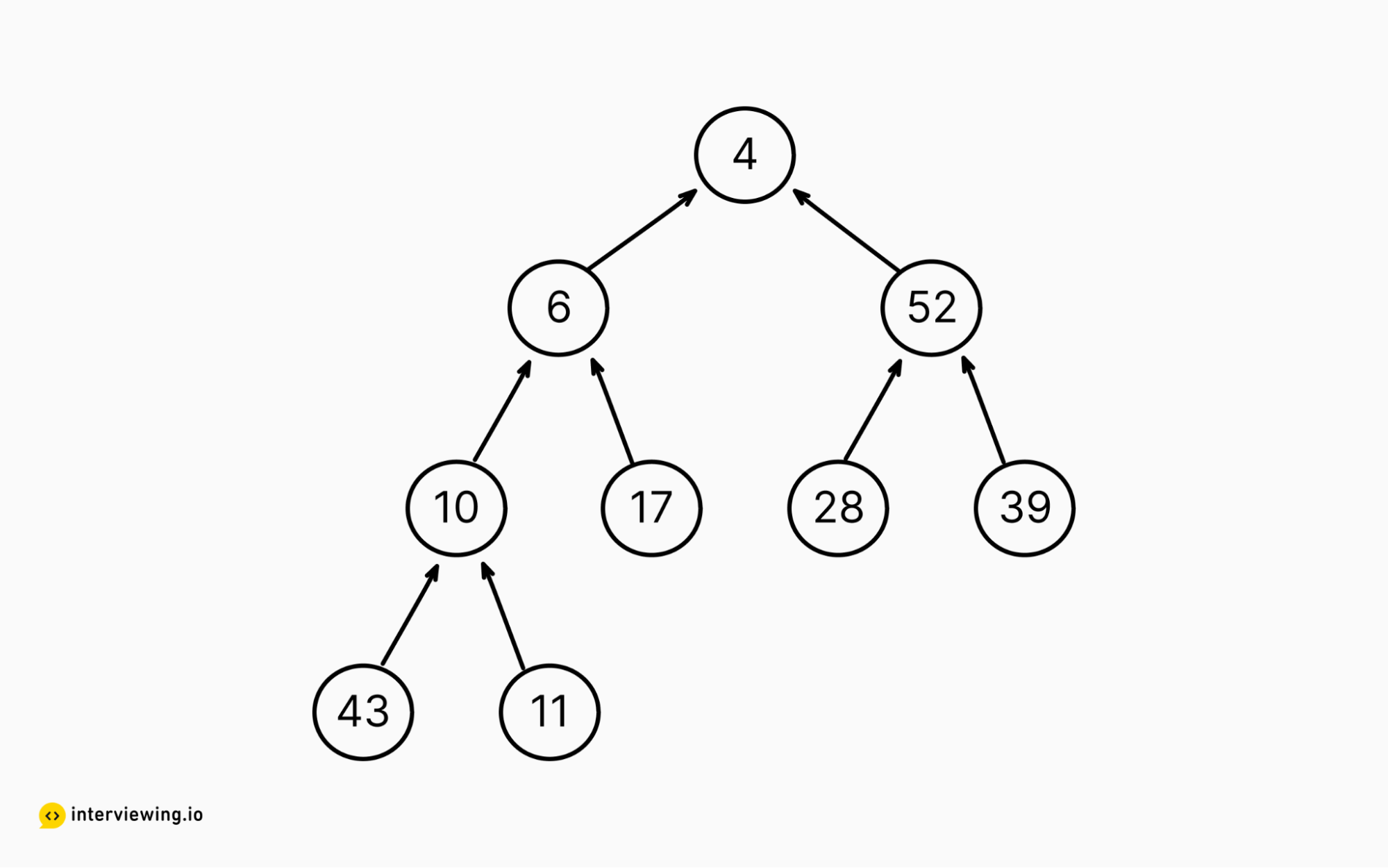Output:

• [52,43,39,17,10,6,28,4,11] (sift up)

OR

• [52,43,39,11,17,28,4,10,6] (sift down)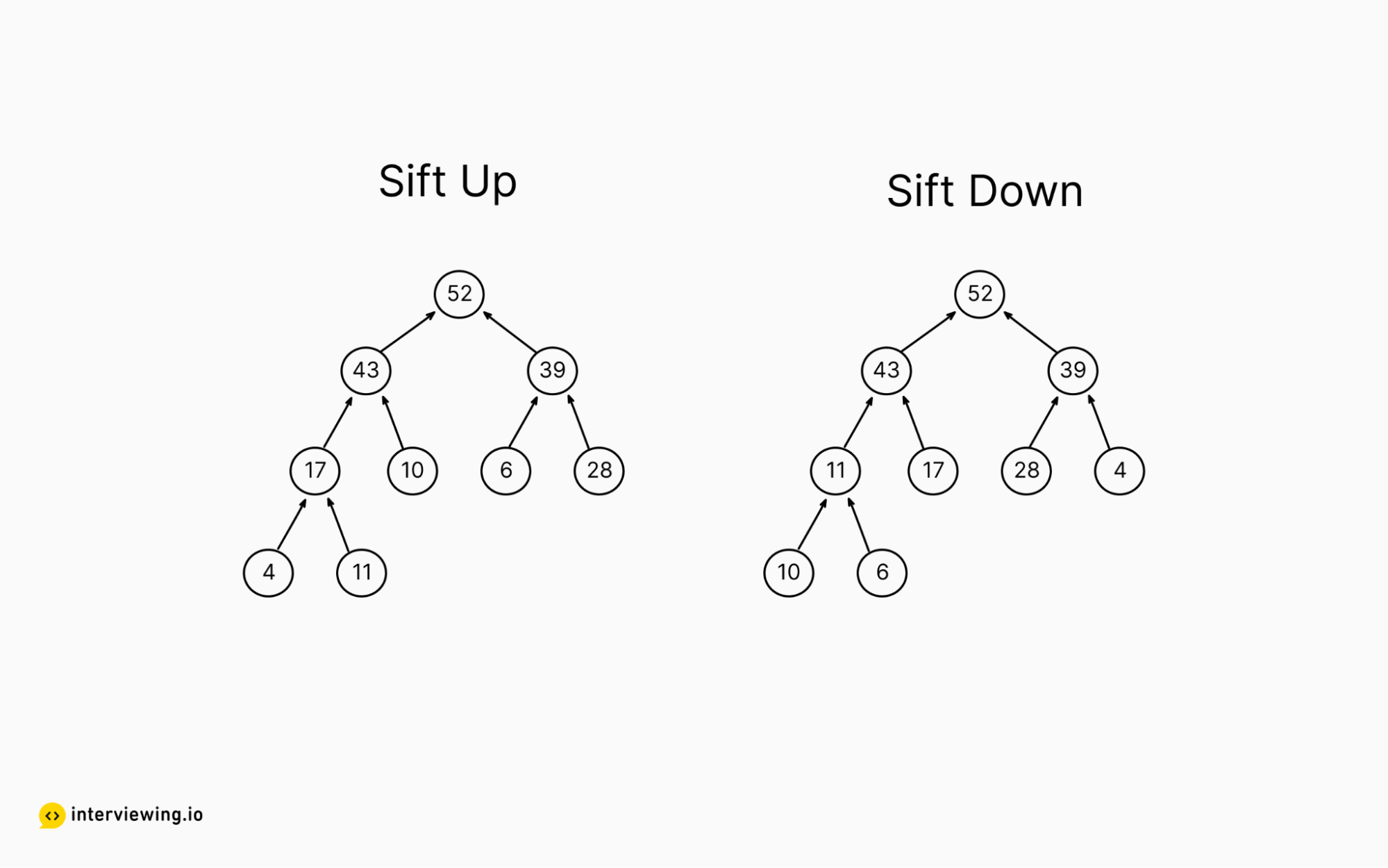Constraints

• `1 <= len(arr) <= 10,000`
• Every integer in the array will be <= 64 bits

## Practice the Build a Max Heap From an Array Problem With Our AI Interviewer

Start AI Interview

## How to Build a Max Heap

The heap data structure is a complete binary tree which satisfies the heap property, meaning each node is at least as large (or small) as each of its children. Max heaps can be encoded in arrays, where:

1. The leftmost element of the array is the largest in the array
2. The children of the node at index `i` are located at `2*i+1` and `2*i+2`

To demonstrate this, consider the following max heap: [7, 5, 6, 4, 2, 1, 3].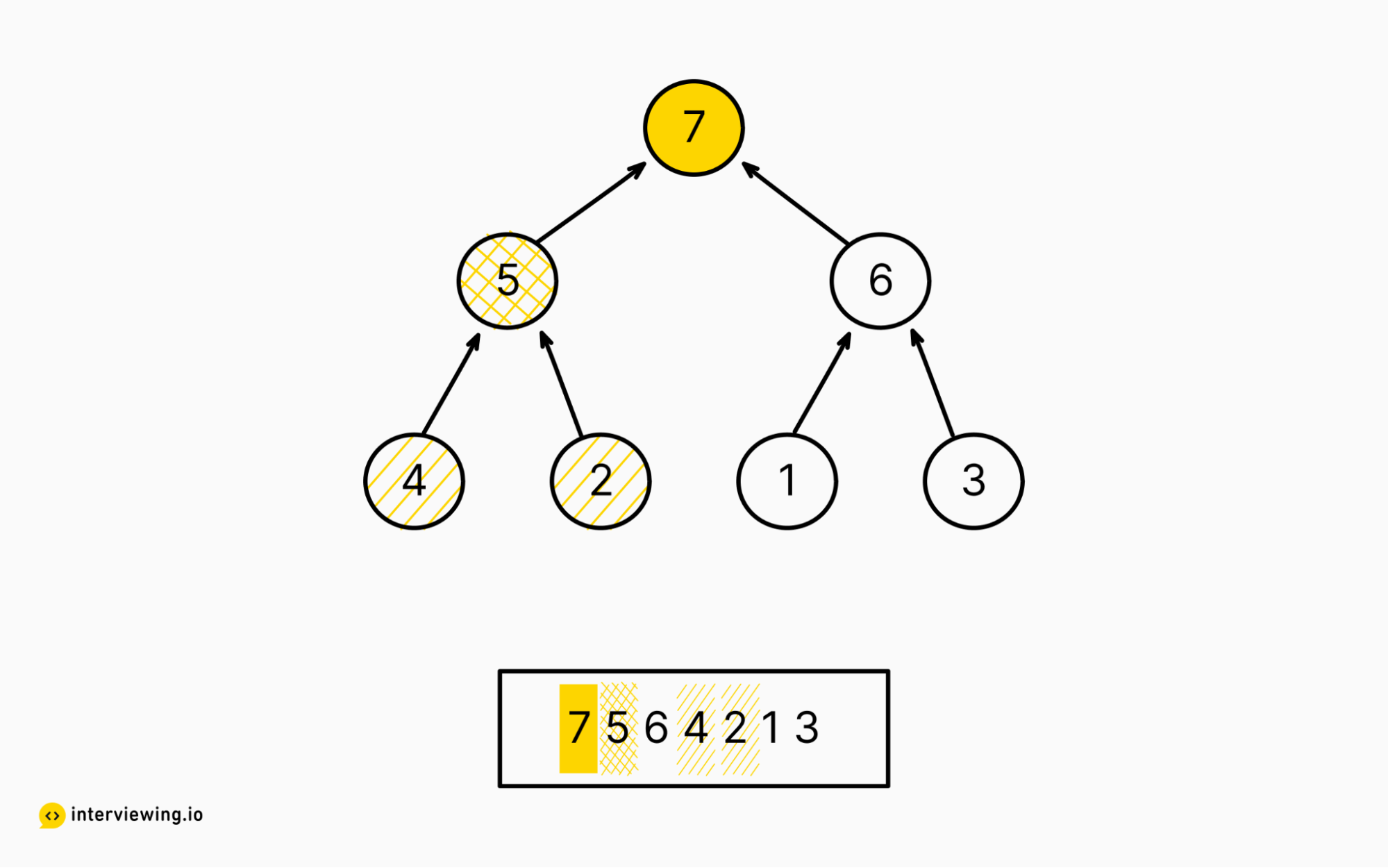Within this array, you can see the two children of `7` (the `0th` index element) at the following indexes: 2 * 0 + 1 = 1, and 2 * 0 + 2 = 2.

Additionally, for `5` (the `1st` index element) you can use the equation again to find its children at indexes: 2 * 1 + 1 = 3, and 2 * 1 + 2 = 4.

Given an arbitrary array, it is possible to reorder the elements such that they satisfy the above properties. A heap sort is a kind of sorting algorithm that creates a priority queue with an array - this structure provides constant time access to the largest element, if performing a max-heapify, or the smallest element, if performing a min-heapify.

Note: A binary heap can be represented as both a binary tree and an array. But, given a collection of integers, there may be many valid orderings that satisfy the min- or max-heap property, which will depend on the algorithm used to “heapify” the array

Two common approaches to “heapifying” an array are to rely on sifting nodes up the tree, and sifting nodes down the tree.

### Approach 1: Sift Up

To sift a node up the tree, we swap a node that is larger than its parent with its parent, and continue doing this (via e.g. recursion ) until it is not larger than its parent.

To solve this problem using the “sift up” approach, we can use algebra to modify the equation we use to find a node’s children. Consider the following equation and note how it can be adjusted to solve for the child node’s and the parent node’s index:

``````child_one_idx = parent_idx * 2 + 1
child_two_idx = parent_idx * 2 + 2
``````

Using algebra to isolate `parent_idx` yields the following:

``````(child_one_idx + 1) / 2 = parent_idx
(child_two_idx + 2) / 2 = parent_idx
``````

Note we now have two separate equations to find the parent index. Specifically, if we use the formula for child one on a node which is the second child, we will end up with a value that is `0.5` larger than the actual parent index. So we need to find one equation that will work in all circumstances, and to accomplish this we can modify our `parent_idx` equation slightly by using Math.floor():

``````parent_index = floor((i - 1 )/ 2)
``````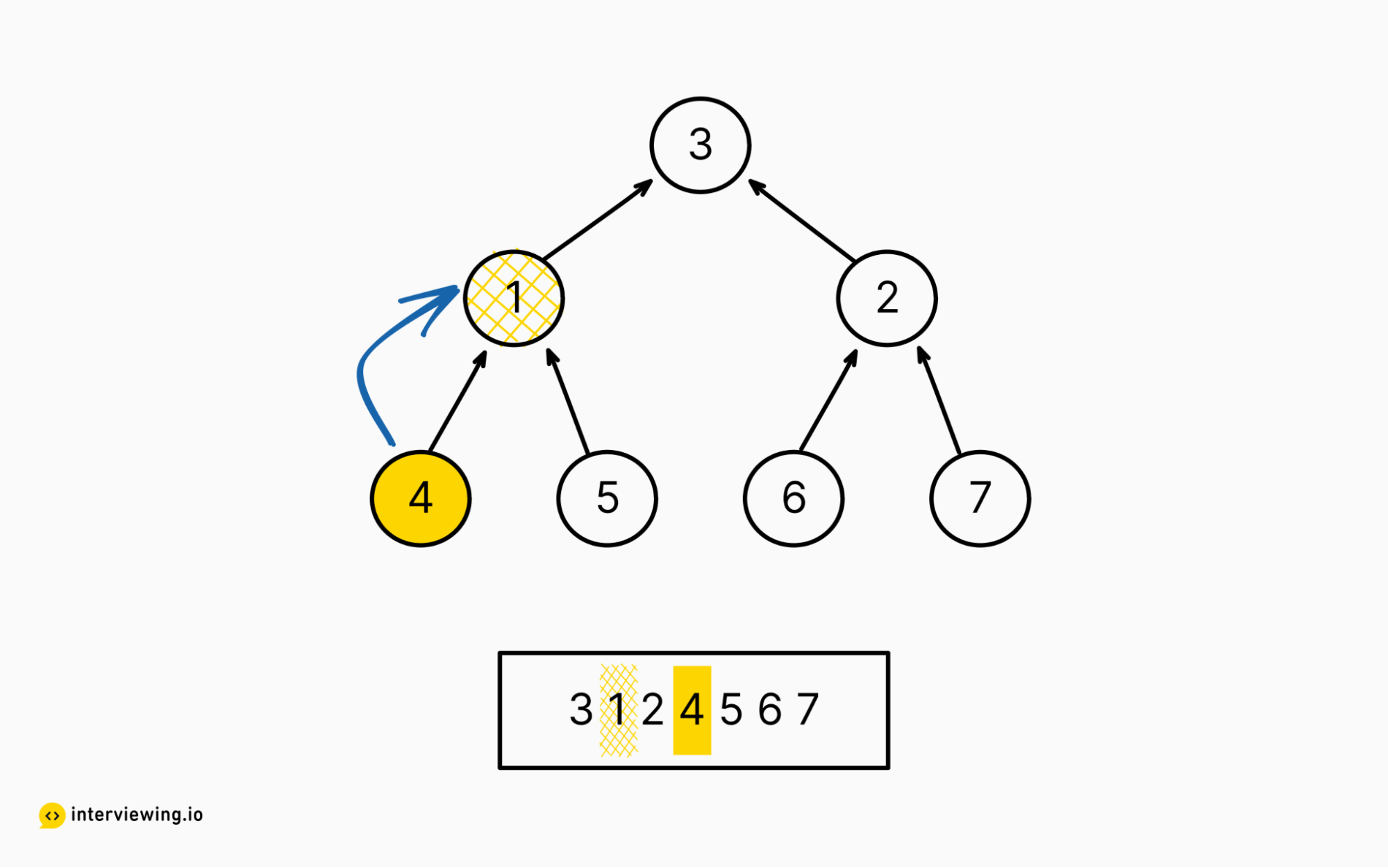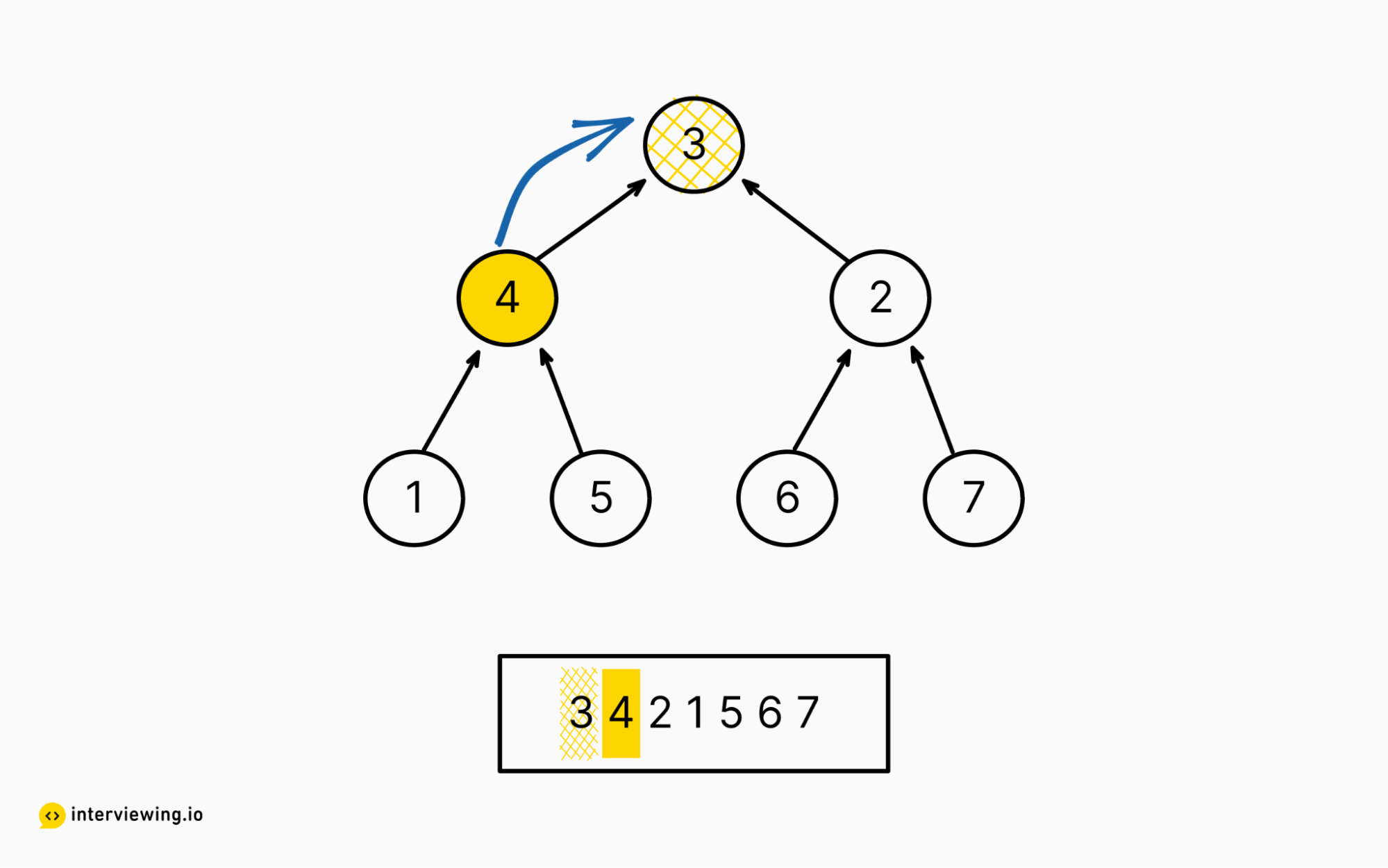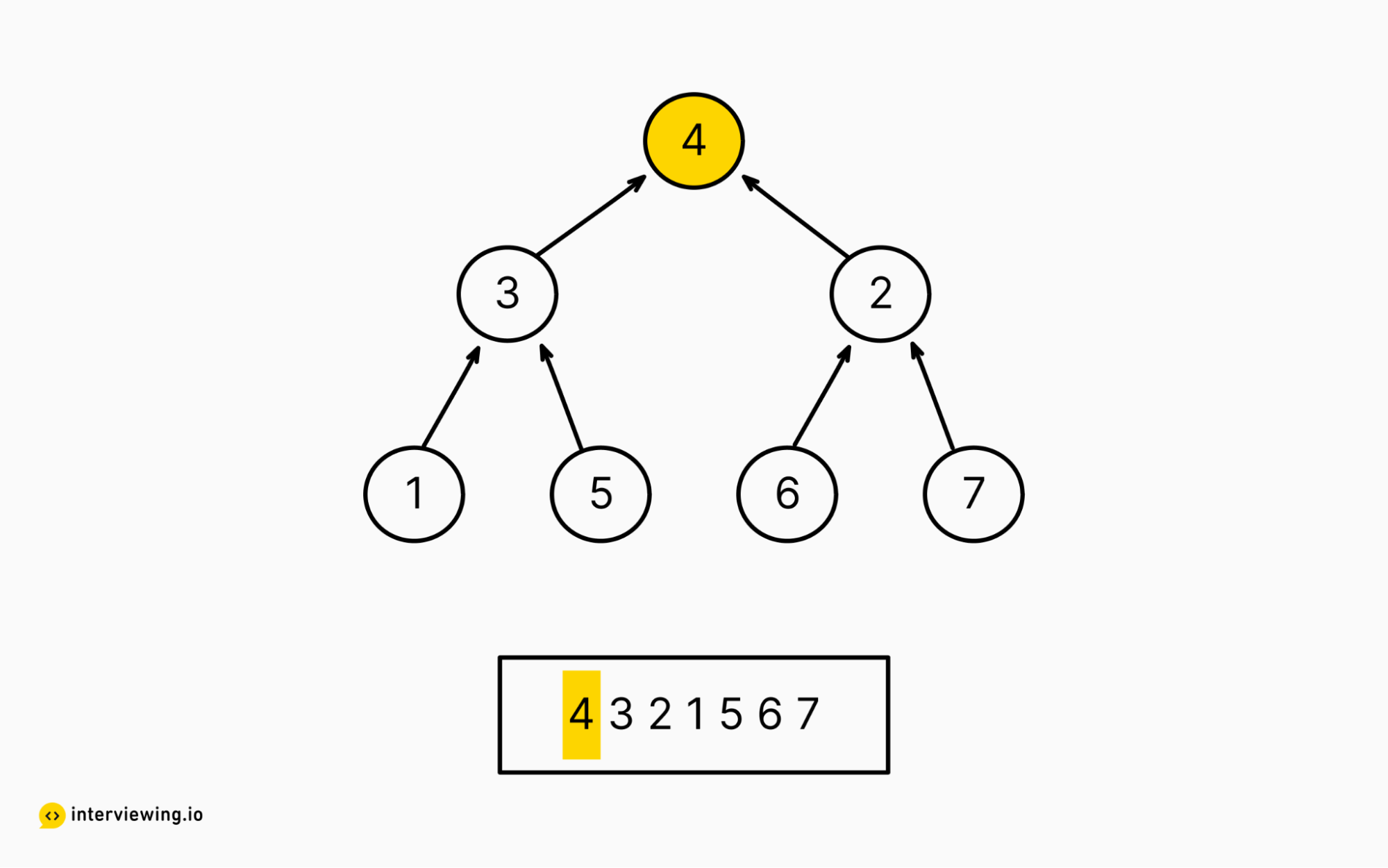To build the heap via sifting up involves starting at the beginning of the tree (head of the array), and iteratively sifting up each node. As the algorithm proceeds, nodes to the left of the current index will form a max heap, until finally all nodes have been sifted up.

``````from math import floor

def build_heap_siftup(arr):
# Skip the first element, since it has no parent
for i in range(1, len(arr)):
siftup(arr, i)

def siftup(arr, index):
if index < 1:
return
parent_idx = floor((index-1)/2)
# is the current node larger than its parent?
if arr[parent_idx] < arr[index]:
# we're larger, swap and check if we're larger than the next parent
swap(arr, parent_idx, index)
siftup(arr, parent_idx)

def swap(arr, first, second):
tmp = arr[first]
arr[first] = arr[second]
arr[second] = tmp``````

#### Time/Space Complexity

• Time complexity: `O(n * log(n))`. This algorithm needs to call siftUp() on all but the root node (the n term) where n is the number of nodes. This runs in linear time, and needs to move each node to the top of the heap (which takes worst-case log(n) time). Hence the O(n * log(n)) time complexity.

### Approach 2: Sift Down

Conversely, we can also “heapify” an array by shifting smaller nodes down the tree.

To sift a node down the tree, we swap a node that is smaller than its children with its largest child, and continue doing so on each subtree with recursion until it is not smaller than its children, or its the last element.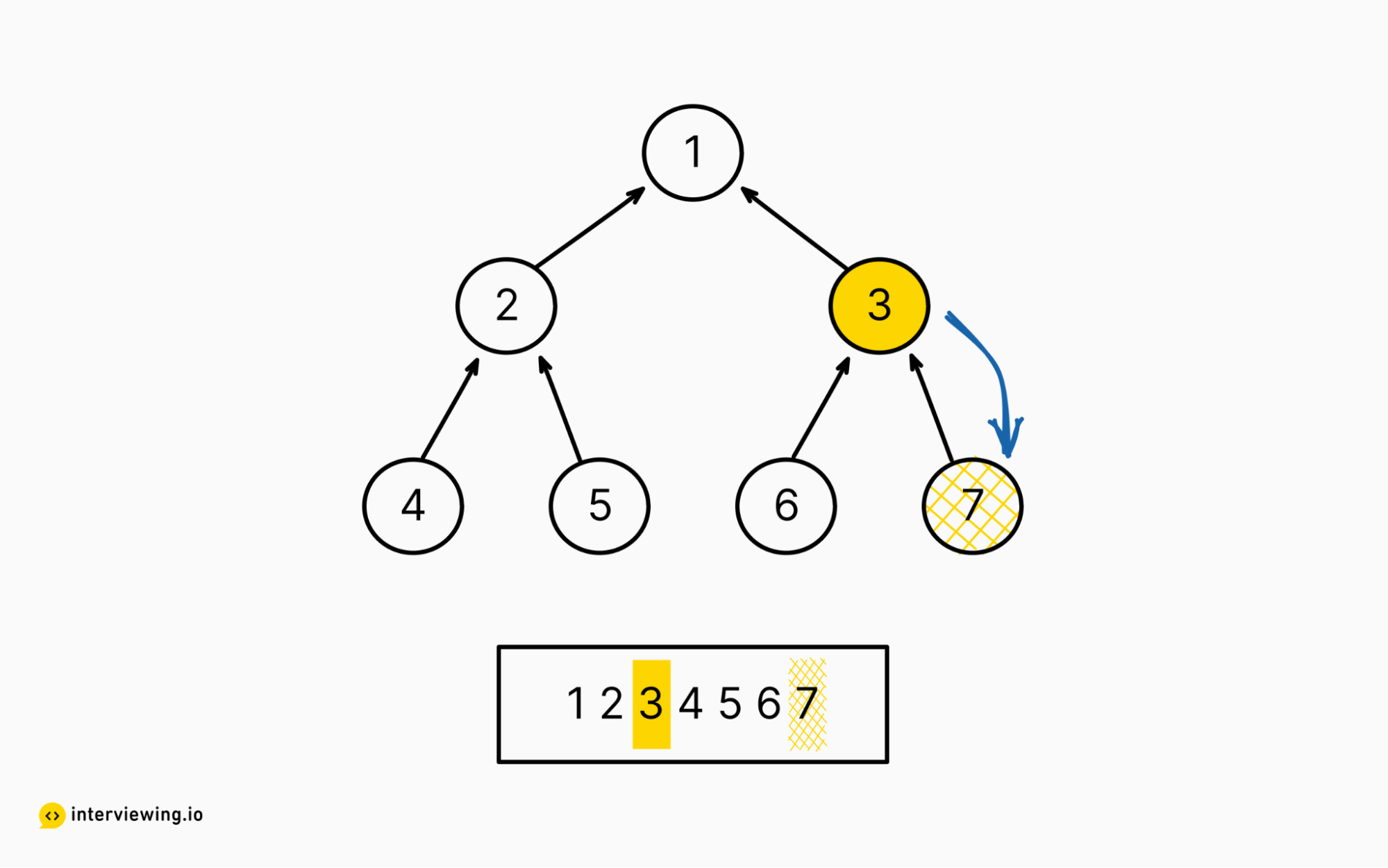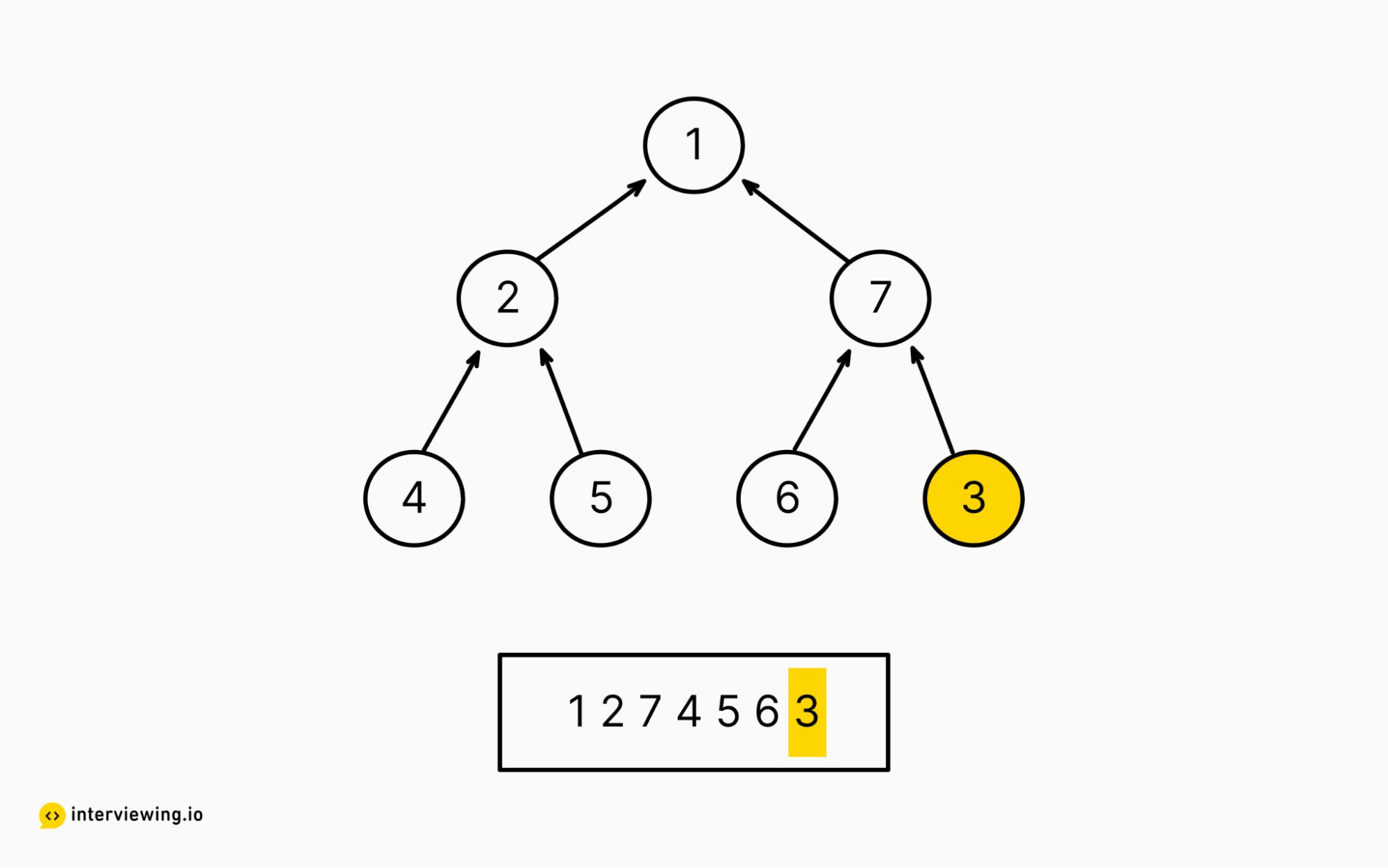To build the heap via sifting down we start at the end and iteratively sifting down each node with the larger of its two children assuming at least one of them is bigger than the node itself. As the algorithm proceeds, nodes to the right of the current index will form max heaps.

Note this approach can also be applied to min heaps by sifting down nodes with children that are smaller than itself.

``````from math import floor

def build_heap_siftdown(arr):
# iterating right to left in the array
# we don't need to start strictly at the end, since those nodes have no children
# floor(len(arr)/2) gets the first node with children in a complete binary tree
for i in range(floor(len(arr)/2), -1, -1):
siftdown(arr, i)

def siftdown(arr, level=0):
if len(arr) <= 1:
return

left_idx = 2*level + 1
right_idx = 2*level + 2
largest = level

# See if the left child is larger
if left_idx < len(arr) and arr[left_idx] > arr[largest]:
largest = left_idx

# See if the right child is larger
if right_idx < len(arr) and arr[right_idx] > arr[largest]:
largest = right_idx

if largest != level:
# There's a child that's larger; switch with the largest child, and
# see if we're smaller than its children
swap(arr, largest, level)
siftdown(arr, largest)

def swap(arr, first, second):
tmp = arr[first]
arr[first] = arr[second]
arr[second] = tmp``````

#### Time/Space Complexity

• Time complexity: `O(n)`
• Space complexity: `O(n)`

To see why the time complexity differs between these two approaches, see this Stack Overflow post.

## Watch Mock Interviews Featuring the Build a Max Heap From an Array Question

interviewing.io is a mock interview platform, and people in our community routinely share their interview replays to help others get better. In the video below, you can watch people solve Max Heap. The interview is in Java. The interviewer is a senior engineer from Google.
Advance this person to the next round?Technical Skills:
3/4
Problem Solving Ability:
1/4
Communication Ability:
3/4

## Build a Max Heap From an Array Frequently Asked Questions (FAQ)

### What's the difference between a max heap and a min heap?

The difference between a max heap and a min heap is in the ordering of the elements. A max heap is a binary tree in which the value of each parent node is greater than or equal to the values of its children. This means that the largest element is always at the root of the tree.

On the other hand, a min heap is a binary tree in which the value of each parent node is less than or equal to the values of its children. This means that the smallest element is always at the root of the tree.

### How do you create a max heap from an array?

There are two approaches you can take: sifting up and sifting down. Sifting up runs in `O(n * log(n))` time. Sifting down runs in `O(n)` time.

When you sift up, you swap a node that is larger than its parent with its parent, and continue doing this (via e.g. recursion) until it is no larger than its parent. When you sift down, you swap a node that is smaller than its children with its largest child, and continue doing so (via e.g. recursion) until it is no smaller than its children.

### How do you build a max heap in linear time?

To build a max heap in linear time, you need to take the “sifting down” approach.